Maths-
General
Easy

Question

# A person invests  Rs10000 for two years at a certain rate of interest compounded annually. At the end of one year, the sum amounts to  Rs12000. Calculate (i). the rate of interest per annum (ii) the amount at the end of the second yearHint:

## The correct answer is: 14400 RUPEES

### Complete step by step solution:(i)We know the formula for total amount = A = P + SI…(i)where A is the total amount, P is the principal amount and SI is simple interest.(for 1st year, both simple interest and compound interest are the same)Here, we have A = 12000 Rs and P = 10000 RsOn substituting the known values in (i), we get SI = 12000 - 10000 = 2000So, we have SI = 2000 RsWe calculate simple interest by the formula,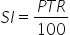…(ii)where P is Principal amount, T is number of years and R is rate of interestHere, we have SI = 2000, P = 10000,T = 1 and R = ?On substituting the known values in (ii), we get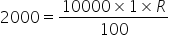Hence the rate of interest  R is 20%.(ii)We know that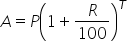…(iii)Here, we have T = 2 years, P = 10000 , R = 20%Amount at the end of second year will be,On substituting the known values in (iii), we get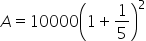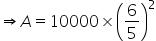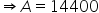Rupees#### With Turito Foundation.#### Get an Expert Advice From Turito.